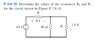# Finding the values of resistors

• ZeeAngel

## Homework Statement

In the problem i have to find the values of resistors using voltage divider, and use KVL to determine the currents.

## Homework Equations

Vi= [Ri / (R1+R2+...+Rn)] * Vs

1/Rtotal = 1/R1 + 1/R2 + ... + 1/Rn

Ʃrise + Ʃdrop = 0

## The Attempt at a Solution

In order for us to use voltage divider the resistors must be in series.

Req = (40*R2)/(40+R2), but we still don't know the resistance of R2.

However, later I tried to use the KVL equation to find the voltage for Req.

-24+8+Veq = 0 → Veq = 16 V

Then I used the voltage divider equation:

Veq = [Req / (R1 + Req) ]* Vs // but again we have two unknowns here again

And that's the point where I stuck, I know this problem is trivial but my brain cannot come up with anything else to solve this.

#### Attachments

•Untitled.png
9.2 KB · Views: 473
Using KVL, we know that Veq=24V-8V=16V. Using Ohm's Law, we can easily get R2=16V/1.6A=10 Ohms. The rest is obvious! :)

Thank you very much :D